• 一、归并排序 1、介绍。 归并排序（MERGE-SORT）是建立在归并操作上的一种有效的排序算法,该算法是采用分治法（Divide and Conquer）的一个非常典型...归并排序是一种稳定的排序方法归并排序是稳定排序，需...
一、归并排序

1、介绍。

归并排序（MERGE-SORT）是建立在归并操作上的一种有效的排序算法,该算法是采用分治法（Divide and Conquer）的一个非常典型的应用。将已有序的子序列合并，得到完全有序的序列；即先使每个子序列有序，再使子序列段间有序。若将两个有序表合并成一个有序表，称为二路归并。归并排序是一种稳定的排序方法。

归并排序是稳定排序，需要额外内存，空间复杂度O(n)。时间复杂度，最佳情况：O(nlogn)  最差情况：O(nlogn)  平均情况：O(nlogn)。

2、步骤。

(1)申请空间，使其大小为两个已经排序序列之和，该空间用来存放合并后的序列。
(2)设定两个指针，最初位置分别为两个已经排序序列的起始位置。
(3)比较两个指针所指向的元素，选择相对小的元素放入到合并空间，并移动指针到下一位置。
(4)重复步骤3直到某一指针超出序列尾，将另一序列剩下的所有元素直接复制到合并序列尾。

3、代码。

public static void main(String[] args) {
System.out.println("------开始------");
//生成生成两份一模一样的随机数组，其中一组用系统自带的方法进行排序，到时候进行验证。
final int number = 100000;
int[] sortArray = new int[number];
int[] sortArrayCopy = new int[number];
for (int i = 0; i < sortArray.length; i++) {
sortArray[i] = (int) (Math.random() * number);
}
System.arraycopy(sortArray, 0, sortArrayCopy, 0, number);//数组复制
Arrays.sort(sortArrayCopy);

//开始排序
long startTime = System.currentTimeMillis();
mergeSort(sortArray);//归并(合并)排序
System.out.println("花费时间：" + (System.currentTimeMillis() - startTime));

//跟系统排序之后数组进行比较，查看是否排序成功。
if (Arrays.equals(sortArray, sortArrayCopy)) {
System.out.println("排序成功");
} else {
System.out.println("排序失败");
}
System.out.println("------结束------");
}

//归并(合并)排序 最佳情况：T(n) = O(n)  最差情况：T(n) = O(nlogn)  平均情况：T(n) = O(nlogn)
private static void mergeSort(int[] array) {
//只剩一个数了
if (array.length < 2) {
return;
}
//将array数组，切成left和right两个数组
int middle = array.length / 2;
int[] left = new int[middle];
int[] right = new int[array.length - middle];
for (int i = 0; i < array.length; i++) {
if (i < middle) {
left[i] = array[i];
} else {
right[i - middle] = array[i];
}
}
//递归
mergeSort(left);
mergeSort(right);
merge(array, left, right);
}

private static void merge(int[] array, int[] left, int[] right) {
int leftIndex = 0, rightIndex = 0;
for (int i = 0; i < array.length; i++) {
if (leftIndex >= left.length) {//left数组已经排完了
array[i] = right[rightIndex++];
} else if (rightIndex >= right.length) {//right数组已经排完了
array[i] = left[leftIndex++];
} else if (left[leftIndex] < right[rightIndex]) {//left数组的值小于right数组的值
array[i] = left[leftIndex++];
} else {//left数组的值大于等于right数组的值
array[i] = right[rightIndex++];
}
}
}

4、结果。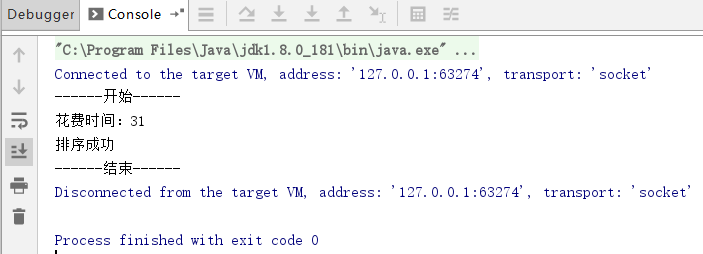二、自然归并排序

1、介绍。

自然归并排序:对于初始给定的数组,通常存在多个长度大于1的已自然排好序的子数组段.例如,若数组a中元素为{4,8,3,7,1,5,6,2},则自然排好序的子数组段有{4,8},{3,7},{1,5,6},{2}.用一次对数组a的线性扫描就足以找出所有这些排好序的子数组段.然后将相邻的排好序的子数组段两两合并,构成更大的排好序的子数组段({3,4,7,8},{1,2,5,6}).继续合并相邻排好序的子数组段,直至整个数组已排好序。

自然归并排序是稳定排序，需要额外内存，空间复杂度O(n)。时间复杂度，最佳情况：O(nlogn)  最差情况：O(nlogn)  平均情况：O(nlogn)。

2、步骤。

跟归并排序的步骤一样，只不过在选择middle的时候，将前面已经排序好的作为一个数组。

3、代码。

public static void main(String[] args) {
System.out.println("------开始------");
//生成生成两份一模一样的随机数组，其中一组用系统自带的方法进行排序，到时候进行验证。
final int number = 100000;
int[] sortArray = new int[number];
int[] sortArrayCopy = new int[number];
for (int i = 0; i < sortArray.length; i++) {
sortArray[i] = (int) (Math.random() * number);
}
System.arraycopy(sortArray, 0, sortArrayCopy, 0, number);//数组复制
Arrays.sort(sortArrayCopy);

//开始排序
long startTime = System.currentTimeMillis();
natureMergeSort(sortArray);//自然归并(合并)排序
System.out.println("花费时间：" + (System.currentTimeMillis() - startTime));

//跟系统排序之后数组进行比较，查看是否排序成功。
if (Arrays.equals(sortArray, sortArrayCopy)) {
System.out.println("排序成功");
} else {
System.out.println("排序失败");
}
System.out.println("------结束------");
}

//自然归并(合并)排序 最佳情况：T(n) = O(n)  最差情况：T(n) = O(nlogn)  平均情况：T(n) = O(nlogn)
private static void natureMergeSort(int[] array) {
//只剩一个数了
if (array.length < 2) {
return;
}
//将array数组，切成left和right两个数组
int middle = array.length / 2;
for (int i = 0; i < array.length - 1; i++) {
if (array[i] > array[i + 1]) {
middle = i;
break;
}
}
int[] left = new int[middle];
int[] right = new int[array.length - middle];
for (int i = 0; i < array.length; i++) {
if (i < middle) {
left[i] = array[i];
} else {
right[i - middle] = array[i];
}
}
//递归
mergeSort(left);
mergeSort(right);
merge(array, left, right);
}

private static void merge(int[] array, int[] left, int[] right) {
int leftIndex = 0, rightIndex = 0;
for (int i = 0; i < array.length; i++) {
if (leftIndex >= left.length) {//left数组已经排完了
array[i] = right[rightIndex++];
} else if (rightIndex >= right.length) {//right数组已经排完了
array[i] = left[leftIndex++];
} else if (left[leftIndex] < right[rightIndex]) {//left数组的值小于right数组的值
array[i] = left[leftIndex++];
} else {//left数组的值大于等于right数组的值
array[i] = right[rightIndex++];
}
}
}

4、结果。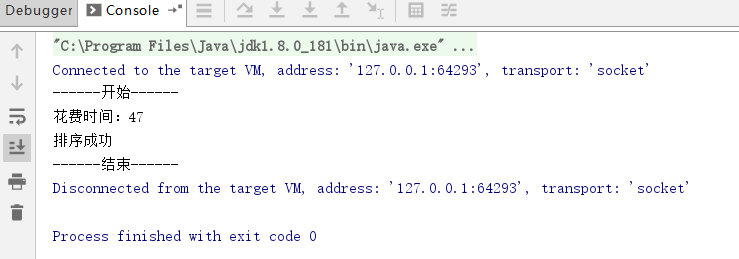展开全文排序算法 合并排序 自然归并算法
• ## 归并排序 详解

万次阅读 多人点赞 2018-05-30 13:38:53
也许有很多同学说，原来也学过很多O（n^2）或者O（n^3）的排序算法，有的可能优化一下能到O（n）的时间复杂度，但是在计算机中都是很快的执行完了，没有看出来算法优化的步骤，那么我想说有可能是你当时使用的测试...
注：内容，图片来自于慕课网liuyubobobo老师的课程。

官方代码链接：https://github.com/liuyubobobo/Play-with-Algorithms

算法复杂度：O（nlogn）；

也许有很多同学说，原来也学过很多O（n^2）或者O（n^3）的排序算法，有的可能优化一下能到O（n）的时间复杂度，但是在计算机中都是很快的执行完了，没有看出来算法优化的步骤，那么我想说有可能是你当时使用的测试用例太小了，我们可以简单的做一下比较：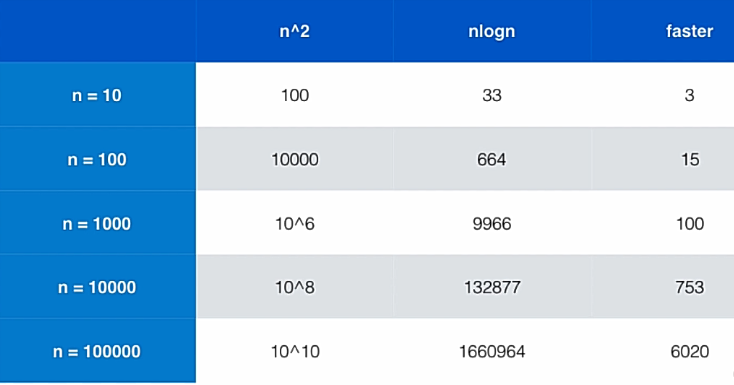当数据量很大的时候 nlogn的优势将会比n^2越来越大，当n=10^5的时候，nlogn的算法要比n^2的算法快6000倍，那么6000倍是什么概念呢，就是如果我们要处理一个数据集，用nlogn的算法要处理一天的话，用n^2的算法将要处理6020天。这就基本相当于是15年。一个优化改进的算法可能比一个比一个笨的算法速度快了许多，这就是为什么我们要学习算法。

核心思想：分治。

下面我们来看归并排序的思路（先讲思路再来具体讲归并的细节）：

归并排序（Merge Sort）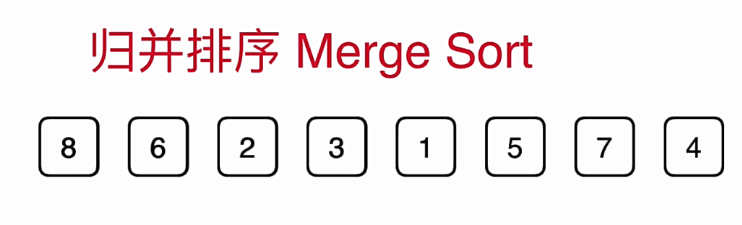当我们要排序这样一个数组的时候，归并排序法首先将这个数组分成一半。如图：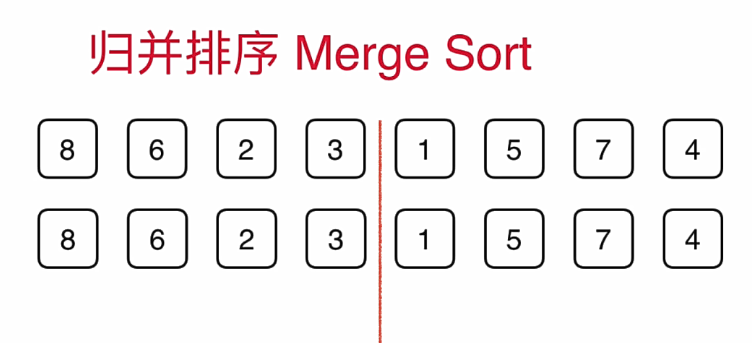然后想办法把左边的数组给排序，右边的数组给排序，之后呢再将它们归并起来。当然了当我们对左边的数组和右边的素组进行排序的时候，再分别将左边的数组和右边的数组分成一半，然后对每一个部分先排序，再归并。如图：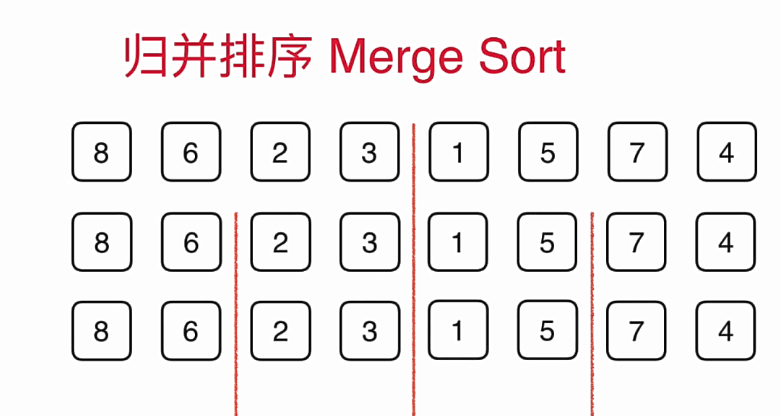对于上面的每一个部分呢，我们依然是先将他们分半，再归并，如图：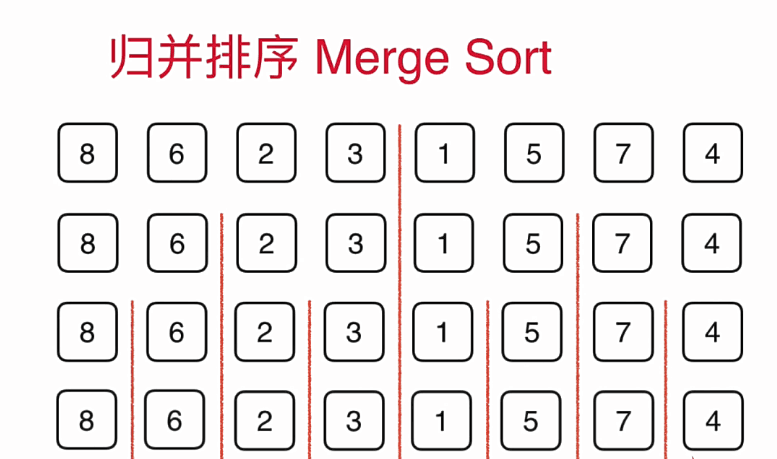分到一定细度的时候，每一个部分就只有一个元素了，那么我们此时不用排序，对他们进行一次简单的归并就好了。如图：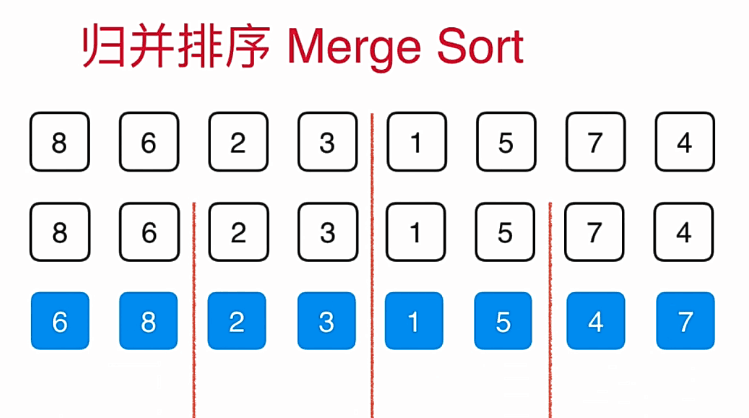归并到上一个层级之后继续归并，归并到更高的层级，如图：直至最后归并完成。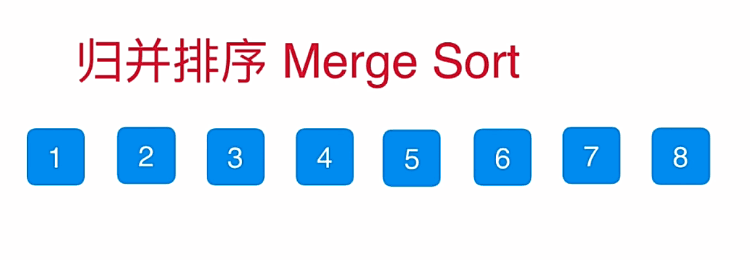那么如何归并呢？我们是否可以用O（n）的算法将两个数组归并到一起形成一个数组呢？如果可以的话，我们将可以用递归的过程来实现整个归并。这是你想起来很简单但是操作起来并不是那么简单的问题。

归并细节：

比如有两个已经排序好的数组，如何将他归并成一个数组？

我们可以开辟一个临时数组来辅助我们的归并。也就是说他比我们插入排序也好，选择排序也好多使用了存储的空间，也就是说他需要o（n）的额外空间来完成这个排序。只不过现在计算机中时间的效率要比空间的效率重要的多。无论是内存也好还是硬盘也好可以存储的数据越来越多，所以设计一个算法，时间复杂度是要优先考虑的。

整体来讲我们要使用三个索引来在数组内进行追踪。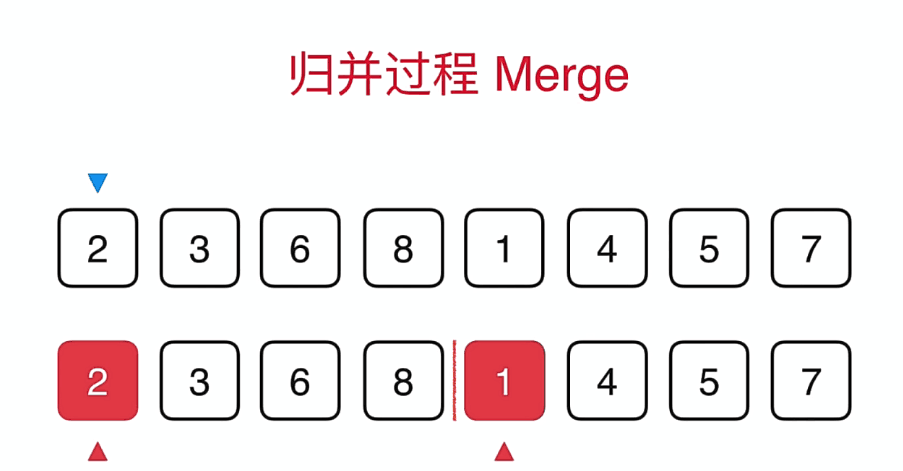蓝色的箭头表示最终选择的位置，而红色的箭头表示两个数组当前要比较的元素，比如当前是2与1比较，1比2小，所以1放到蓝色的箭头中，蓝色的箭头后移，1的箭头后移。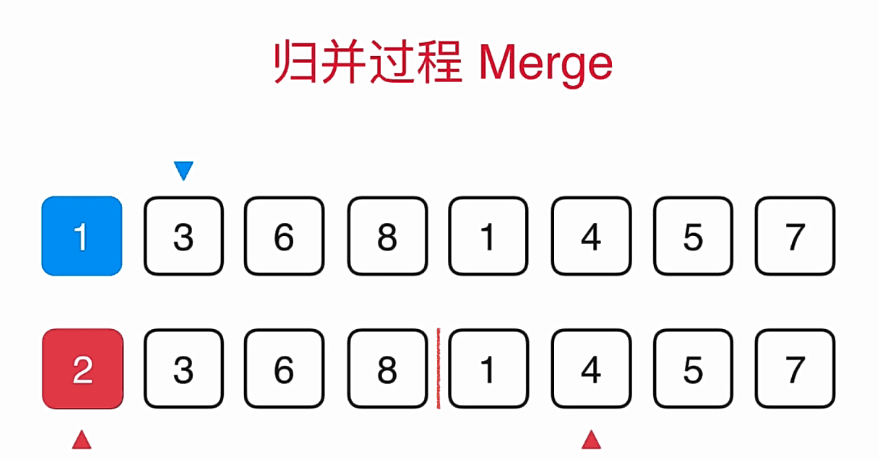然后2与4比较，2比4小那么2到蓝色的箭头中，蓝色箭头后移，2后移，继续比较.......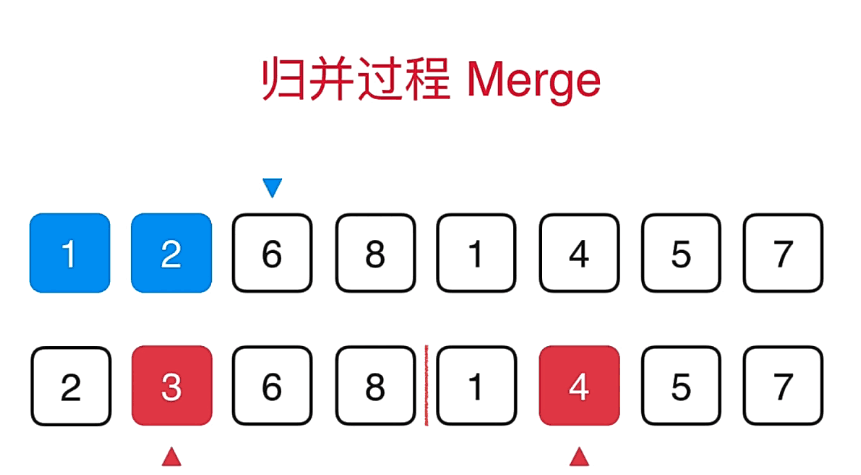归并思路就是这样了，最后唯一需要注意的是那个先比较完的话，那么剩下的直接不需要比较，把后面的直接移上去就可以了，这个需要提前判定一下。

归并排序代码如下：

#include<cstdio>
#include<iostream>
#include<algorithm>
#include<cstring>
using namespace std;

void merge(int a[],int l,int r,int mid)
{
int aux[r-l+1],i,j,k;

for(k=l;k<=r;k++)
aux[k-l]=a[k];

i=l;
j=mid+1;
for(k=l;k<=r;k++)
{
if(i>mid)
{
a[k]=aux[j-l];
j++;
}
else if(j>r)
{
a[k]=aux[i-l];
i++;
}
else if(aux[i-l]>aux[j-l])
{
a[k]=aux[j-l];
j++;
}
else
{
a[k]=aux[i-l];
i++;
}

}

}

void merge_sort(int a[],int l,int r)
{
if(l>=r)
return ;

int mid=(l+r)/2;

merge_sort(a,l,mid);
merge_sort(a,mid+1,r);
merge(a,l,r,mid);

}

void mergesort(int a[],int l,int r)
{
merge_sort(a,l,r-1);
}

int main()
{
int a,n,i;
scanf("%d",&n);

for(i=0;i<n;i++)
scanf("%d",&a[i]);

mergesort(a,0,n);

for(i=0;i<n;i++)
printf("%d ",a[i]);

return 0;
} 


展开全文• ## 归并排序

千次阅读 2019-03-01 11:31:48
归并排序
0.时间复杂度
lgn * n

1.Java实现
    private static void println(int[] array) {
for (int item : array) {
System.out.print(item + " ");
}
System.out.println();
}

private static void mergeSortByIndex(int[] array, int startIndex, int endIndex) {
// 最小处理块1 然后依次往上层归并
if (startIndex < endIndex) {
int centerIndex = (startIndex + endIndex) / 2;
mergeSortByIndex(array, startIndex, centerIndex);
mergeSortByIndex(array, centerIndex + 1, endIndex);
mergeArray(array, startIndex, centerIndex, endIndex);
}
}

private static void mergeArray(int[] array, int startIndex, int centerIndex, int endIndex) {
int[] tempArray = new int[endIndex - startIndex + 1];
int leftIndex = startIndex;
int rightIndex = centerIndex + 1;
boolean leftFlag = true;
boolean rightFlag = true;
int temp = 0;
while (temp <= endIndex - startIndex) {
if (leftIndex > centerIndex) {
leftFlag = false;
}
if (rightIndex > endIndex) {
rightFlag = false;
}
if (leftFlag && rightFlag) {
// 左右2边都有
//                if (array[leftIndex] <= array[rightIndex]) {
if (array[leftIndex] >= array[rightIndex]) {
tempArray[temp] = array[leftIndex];
leftIndex ++;
} else {
tempArray[temp] = array[rightIndex];
rightIndex ++;
}
} else if (!leftFlag && !rightFlag) {
// 左右2边都没有
break;
} else if (!leftFlag && rightFlag) {
// 只有右边有
tempArray[temp] = array[rightIndex];
rightIndex ++;
} else {
// 只有左边有
tempArray[temp] = array[leftIndex];
leftIndex ++;
}
temp ++;
}

// 复制到原数组中
for (int copyIndex  = 0; copyIndex  < tempArray.length; copyIndex ++) {
array[startIndex + copyIndex] = tempArray[copyIndex];
}
}

private static void mergeSort(int[] array) {
mergeSortByIndex(array, 0, array.length - 1);
}

public static void main(String[] args) throws Exception {
//        int[] array = {10, 9, 8};
int[] array = {8, 9, 10};
println(array);
mergeSort(array);
println(array);
}



展开全文• C语言实现归并排序 文章目录C语言实现归并排序2路归并排序算法1.定义动态数组2.初始化动态数组3.归并操作4.归并排序算法实现项目完整代码运行效果图 2路归并排序算法 1.定义动态数组 //定义一个动态数组 typedef ...
C语言实现归并排序
文章目录C语言实现归并排序2路归并排序算法1.定义动态数组2.初始化动态数组3.归并操作4.归并排序算法实现项目完整代码运行效果图
2路归并排序算法
1.定义动态数组
//定义一个动态数组
typedef struct {
int *data;
} DSqList;

2.初始化动态数组
//动态数组初始化
void InitDSqList(DSqList &L, int len) {
//根据数组A的长度动态分配辅助数组B的空间
L.data = (int *) malloc(len * sizeof(int));
}

3.归并操作
//归并操作——表A中的两个子表A[low...mid]与A[mid+1...high]各自有序，将它们合并为一个有序表
void Merge(int A[], int low, int mid, int high) {
int i, j, k;

for (int p = low; p <= high; ++p) {     //将数组A中的元素对应复制到辅助数组B中
B.data[p] = A[p];
}
for (i = low, j = mid + 1, k = i; i <= mid && j <= high; ++k) {
if (B.data[i] <= B.data[j])     //比较辅助数组B左右两段子表中的元素，并将较小的值复制到A中相应位置
A[k] = B.data[i++];
else
A[k] = B.data[j++];
}
while (i <= mid)                    //如果左子表未检查完，将剩余元素依次复制到A中
A[k++] = B.data[i++];
while (j <= high)                   //如果右子表未检查完，将剩余元素依次复制到A
A[k++] = B.data[j++];
}

4.归并排序算法实现
//归并排序
void MergeSort(int A[], int low, int high) {
if (low < high) {
int mid = (low + high) / 2;         //从中间进行划分，分成两个子表
MergeSort(A, low, mid);             //递归的对左边的子表进行归并排序
MergeSort(A, mid + 1, high);    //递归的对右边的子表进行归并排序
Merge(A, low, mid, high);           //将左右子表进行归并
}
}

项目完整代码
//归并排序（稳定，空间效率为O(n)，时间效率为O(nlogn)）
#include <stdio.h>
#include <stdlib.h>

//定义一个动态数组
typedef struct {
int *data;
} DSqList;

//声明一个全局动态辅助数组B
DSqList B;

//动态数组初始化
void InitDSqList(DSqList &L, int len) {
//根据数组A的长度动态分配辅助数组B的空间
L.data = (int *) malloc(len * sizeof(int));
}

//归并操作——表A中的两个子表A[low...mid]与A[mid+1...high]各自有序，将它们合并为一个有序表
void Merge(int A[], int low, int mid, int high) {
int i, j, k;

for (int p = low; p <= high; ++p) {     //将数组A中的元素对应复制到辅助数组B中
B.data[p] = A[p];
}
for (i = low, j = mid + 1, k = i; i <= mid && j <= high; ++k) {
if (B.data[i] <= B.data[j])     //比较辅助数组B左右两段子表中的元素，并将较小的值复制到A中相应位置
A[k] = B.data[i++];
else
A[k] = B.data[j++];
}
while (i <= mid)                    //如果左子表未检查完，将剩余元素依次复制到A中
A[k++] = B.data[i++];
while (j <= high)                   //如果右子表未检查完，将剩余元素依次复制到A
A[k++] = B.data[j++];
}

//归并排序
void MergeSort(int A[], int low, int high) {
if (low < high) {
int mid = (low + high) / 2;         //从中间进行划分，分成两个子表
MergeSort(A, low, mid);             //递归的对左边的子表进行归并排序
MergeSort(A, mid + 1, high);    //递归的对右边的子表进行归并排序
Merge(A, low, mid, high);           //将左右子表进行归并
}
}

int main() {
int A[] = {49, 38, 65, 97, 76, 13, 27};
int len = sizeof(A) / sizeof(int);

//初始化辅助数组B
InitDSqList(B, len);

//归并排序
MergeSort(A, 0, len - 1);
//输出排序后的结果
printf("归并排序后的结果为：");
for (int i = 0; i < len; ++i) {
printf("%d ", A[i]);
}
return 0;
}

运行效果图
    int A[] = {49, 38, 65, 97, 76, 13, 27};展开全文C语言
• 归并排序（merge sorting）是利用归并的思想实现的排序方法，该算法采用经典的分治（divide and conquer）策略。分治策略将问题分解（divide）分解为一些小的问题然后递归求解，而治（conquer）的阶段将分的阶段...归并算法 java 算法 合并排序
• 归并排序算法一、归并排序的概念二、原地归并的抽象方法（一）、原地归并的抽象方法的概念（二）、原地归并的抽象方法的代码示例三、自顶向下的归并排序（一）、自顶向下的归并排序的概念（二）、自顶向下的归并排序...链表 算法 数据结构 java...